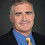## Thursday, December 07, 2017

### Razor's Edge

Informally the sensitivity conjecture asks whether every hard Boolean function has a razor's edge input, where flipping a random bit has a reasonable chance of flipping the output.

Let's be more precise. We consider functions f mapping {0,1}n to {0,1}. For every input x, the decision tree complexity at x is the least number of bits of  x you would need to query to decide whether the function outputs 0 or 1. The decision tree complexity of a function is the maximum decision tree complexity over all possible x. Most interesting functions have high decision tree complexity, even the lowly OR function requires querying every bit on the input of all zeroes. The decision tree complexity is polynomially-equivalent to randomized-complexity, quantum complexity, certificate complexity, and the degree of a polynomial that computes the function exactly or approximately. The recent paper by Aaronson, Ben-David and Kothari gives a nice chart showing the relationship between these measures and references to the various papers giving the bounds.

The sensitivity of f on an input x is the number of bit locations i such that f(x)≠f(x⊕i) where x⊕i is x with the ith bit flipped. The sensitivity of f is the maximum sensitivity over all inputs. The sensitivity conjecture states that there is some ε>0 such that the sensitivity of f is at least mε if the decision tree complexity is at least m. If the conjecture were true then for any function with maximal decision tree complexity n (querying every input bit) there must be some razor's edge input x such that flipping a random bit of x has probability at least n of flipping the output.

I find it surprising that we have no proof or counterexample to this purely combinatorial question. There is a generalization of sensitivity known as block sensitivity which is the largest set of disjoint blocks where flipping the bits in any block flips the output bit. Block sensitivity is known to be polynomially related to decision tree complexity.

In a future post I'll talk about some approaches towards resolving this conjecture.

1.A small typo: I think you mean that the probability of flipping the output is n to the minus epsilon.

2.Thanks. Fixed.

3.What's known about how small ε would have to be? I think at n=4 we can see that it is at most log_3(2).

4.This comment has been removed by the author.